UP TO 15 % DISCOUNT

Get Your Assignment Completed At Lower Prices

Plagiarism Free Solutions
100% Original Work
24*7 Online Assistance
Native PhD Experts
Hire a Writer Now
MA4814 Computational Fluid Dynamics Assignment, NUS, Singapore Apply the finite volume technique to discretize and solve one-dimensional fully developed laminar
 University National University of Singapore (NUS) Subject Computational Fluid Dynamics
Posted on: 6th Sep 2023

# MA4814 Computational Fluid Dynamics Assignment, NUS, Singapore Apply the finite volume technique to discretize and solve one-dimensional fully developed laminar

• Learning Objectives

•  Determine the criterion for grid convergence for a laminar one-dimensional channel flow
•  Solve nonlinear one-dimensional channel flow for non-Newtonian fluid

Problem 1

Apply the finite volume technique to discretize and solve one-dimensional fully developed laminar flow between two horizontal parallel plates governed by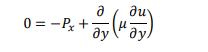𝑢𝑢 is the velocity, 𝑃𝑃𝑥𝑥 is the pressure gradient and µ is the viscosity. In conservative form, this can be written as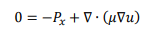##### Hire a Professional Essay & Assignment Writer for completing your Academic Assessments

• A sketch for the cells, clearly marking faces and nodes for internal and boundary cells.
• Apply the linear approximation and use Dirichlet (velocity specified) boundary conditions to determine the approximate equations for internal and boundary cells.
• Compute the velocity distribution and compare your result with the exact solution, by adapting one of the uploaded codes. The number of grid cells is left up to you to determine. The solution must be grid converged.
For the numerical solution, let 𝑃𝑃𝑥𝑥 = 2µ, ℎ = 0.1, 𝑢𝑢(0) = 𝑢𝑢1 = 0.01, & 𝑢𝑢(ℎ) = 𝑢𝑢2 = 0. For grid convergence, you may define an error norm , and require that the error is less than 0.01𝑢 = 0.01 × 0.01. The exact solution is given by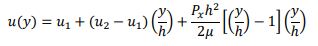Problem 2

Apply the finite volume technique to discretize and solve one-dimensional fully developed laminar non-Newtonian flow between two horizontal parallel plates governed by,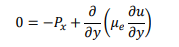𝑢𝑢 is the velocity, 𝑃𝑃𝑥𝑥 is the pressure gradient. For non-Newtonian fluids, the viscosity µ𝑒𝑒 depends on the flow strain rate, which for one-dimensional fully developed flow is approximated by,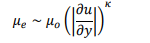where 𝜇𝜇𝑜𝑜, 𝜅𝜅 are constants. Non-Newtonian fluids exist in several important applications, particularly in fluids using in printing, molten plastics used in 3D printers and most important for us, in blood and rheological flows. For more insight, you can check https://www.rheosense.com/applications/viscosity/newtonian-non-newtonian to learn more about
the shear thinning and thickening effects. As this is a non-linear problem, it is highly recommended

Get Help By Expert

Categories:-
Tags:- MA4814 NUS##### No Need To Pay Extra
• Turnitin Report

\$10.00

\$9.00
Per Page
• Consultation with Expert

\$35.00
Per Hour
• Live Session 1-on-1

\$40.00
Per 30 min.
• Quality Check

\$25.00
##### Free

New Special Offer

## Get 30% Off

UP TO 15 % DISCOUNT

Get Your Assignment Completed At Lower Prices

Plagiarism Free Solutions
100% Original Work
24*7 Online Assistance
Native PhD Experts
Hire a Writer Now
My Assignment Help SG Services
My Assignment Help SG